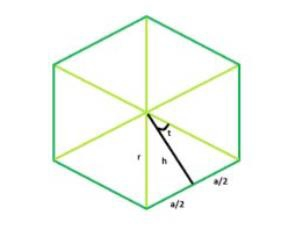# Area of a n-sided regular polygon with given Radius in C Program?

A polygon is a ‘n’ sided closed figure.N sided polygon means a polygon with n equal sides. The radius of a polygon is distance between center and vertex.

In the figure we can see that the whole polygon can be divided into n equal polygon

We know,

area of the triangle = (base * height)/2

Area of the small triangle using trigonometric logic,

area = r2*sin(t)cos(t) = (r2*sin(2t))/2

So, area of the polygon:

Area = n * (area of one triangle)

= n*r2*sin(2t)/2
= n*r2*sin(360/n)/2## Example

#include <stdio.h>
#include <math.h>
int main() {
float r = 4 n = 12;
float area = ((r * r * n) * sin((360 / n) * 3.14159 / 180)) / 2;
printf("area = %f", area);
return 0;
}

## Output

area = 47.999962